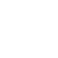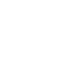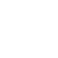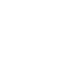# Help! How do I calculate how much flooring I need?

If you’ve been trying to calculate how much flooring you need and coming up baffled (or frustrated) don’t worry. When it comes to flooring products, there are a lot of confusing terms to get your head around, and lots of conflicting information online.

We’re here to help you figure out exactly how much flooring you need and the type of measurements you need to take. From here, you will be able to calculate the approximate cost of your flooring based on whichever products you’re choosing.

In this guide, we will go through the following points:

## Types of flooring measurement

There are two types of flooring measurement you will come across: linear (or lineal) metre, and square metre.

• Linear metre typically refers to the measurements of how carpet is sold.
• Laminate, timber and vinyl flooring is typically sold in amounts of square metres.

## Measurements you need to be familiar with

### 1- Linear (or lineal) metre

A linear or lineal metre measures length. It is how carpet rolls are measured, and these are usually 3.66m wide (unless stated otherwise). Depending on the size of your room, you will calculate how much length (linear metre) of product you require from a carpet roll.

### How to measure a linear (lineal) metre?

A roll of carpet is (typically) 3.66m wide, so the length of carpet you need will be based on the measurements of your room. A commonly used calculation is to measure the square metres of your space and then divide that by 3.66m to determine the linear metres. However, this method is not always accurate, especially if the room is less than 3.66m long.

### 2- Square metre

A square metre (m2) is a unit of measurement of an area. It is a square whose sides measure exactly one metre.

### How to measure a square metre

Breakdown larger spaces into rectangles to make measuring easier. Then, measure each rectangle by length and width with a sturdy, accurate measuring tape. Next, multiply the length and width together to determine how many square metres are in that space. For example, if your room is 6m long by 4m wide, 6m x 4m = 24 square metres (24m2).

### Converting linear (lineal) metre to square metre

Multiply the length of the linear metre by the width. For carpet rolls, this is usually 3.66m. That would mean a carpet roll that is 8 linear metres would be calculated as 8m x 3.66m = 29.28 square metres (29.28m2).

## How to calculate the amount of flooring you need?

### Before you start!

Start by deciding what type of flooring you would like, whether it be carpet, tile, vinyl, laminate or timber.

Next, measure the floor space of your room(s). For extra help on how to do this, we’ve got you covered in this room measurement guide. A six metre by four metre room (6.0m x 4.0m) equates to 24 square metres (24m2).

We recommend that you round up your measurements to ensure you have enough materials to finish your flooring project. A good rule of thumb is to add 5-10% extra to your calculations, depending on the type of flooring you choose. For timber flooring in a room that is 24m2, you would add 5% which is 1.2m extra, for a total of 25.2 of product and for carpet you would add 10%, making a total of 26.4m.

### Calculating laminate, vinyl or timber flooring per square metre

Calculate the square metres of your floorspace (using the above method) including your additional wastage amount of 5-10%.
Then, check the product type. If it comes in ‘packs,’ you will be able to calculate the number of packs you need to meet the amount of square metres you must cover. For example, our Duchess planks come in a pack of 1.624m2.  If your room is 24m2, you would purchase 16 packs to ensure you have 25.2m2 worth of laminate planks (24m2 plus an additional 5% for wastage/safety).

### Calculating carpet per linear (lineal) metre

Calculating the linear metres needed to carpet your space is a bit more tricky. It can depend on the width of the room. For example, if you have a room that is 3m x 3m the total square metres is 9.

If you use the formula of adding 10% then dividing this by the width of the carpet roll (3.66m), the result is 2.7m which is not enough length for a 3m long room. In some cases this commonly used formula can be successful when calculating the linear metres required for a room that is wider than the carpet roll.

### How much carpet do I need?

We highly recommend that you calculate the square metres of the room or rooms to be carpeted and get a carpeting  professional to recommend how much you exactly need.

In calculating the amount of carpet required, a professional  will take into account any joins that may be needed for spaces that are wider than the carpet roll. They will ensure that joins and wastage are kept to a minimum by determining the most efficient way to cover your space. Miscalculations can result in excessive wastage which can become costly especially if  you have multiple rooms to carpet.

### How much timber, laminate or vinyl planks do I need?

You need to cover the floor space area, calculated in metres squared (using the above method) plus an additional 5% extra to cover any mishaps (or mis-calculations) for installation.

See our flooring specialists to find out exactly how much floorcovering you need. If you have a house plan or can draw your room with dimensions then the job of our Flooring Solution Specialists is a lot easier and more accurate..

2020-12-11T12:29:44+08:00

### About the Author: Ian MinottiIan is a renowned floor covering expert in product knowledge and installation. He is specialized in all types of floor coverings for many years and his knowledge in the industry is exceptional. Feel free to ask Ian any floor covering question you may have.

#### Our Products